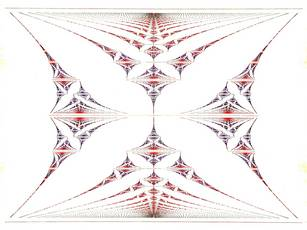### Operator Algebras and Noncommutative GeometryThe spectrum of the canonical operator in the noncommutative 2-torus Tθ depending on the parameter θ ∈ [0,1]© Douglas Hofstadter's butterfly. Licensed under Creative Commons Attribution-Share Alike 3.0 via Wikimedia Commons http://commons.wikimedia.org/wiki/File:Gplot_by_Hofstadter.jpg

An operator algebra is an algebra of continuous linear operators on a Hilbert space. Such algebras can be associated to a variety of problems in mathematics and mathematical physics. The study of operator algebras is based on methods from analysis, algebra, and algebraic topology. Topics of particular interest are the theory of C*-algebras and their classification, K-theory of operator algebras, von Neumann algebras, (C*)-dynamical systems, representation theory of locally compact groups, noncommutative geometry, and mathematical physics.

The specialization Operator Algebras has strong connections to topology, but there are also links to other areas of mathematics like algebra and number theory, differential geometry, partial differential equations, probability, and geometric group theory. Some knowledge in any of these fields is therefore quite useful.

# Prerequisites

A course on Functional Analysis with the following content:
Hahn-Banach theorems, weak and weak-*-topologies, operators on Banach and Hilbert spaces, spectral theorem for compact operators on Hilbert spaces.

# Courses for the specialisation in Operator Algebras and Noncommutative Geometry

Winter semester 2020/2021

Prof. Dr. Wilhelm Winter: Operator algebras (Type I)

Summer semester 2021

Prof. Dr. Wilhelm Winter: Operator algebras II (Type I, II)